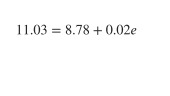PSABM5UM - Practice---Acc7.5 Lesson 3 Comparing Proportional Relationships (8.EE.B, 8.EE.B.5)
Part A)

A contractor must haul a large amount of dirt to a work site. She collected information from two hauling companies. EZ Excavation gives its prices in a table. Happy Hauling Service gives its prices in a graph.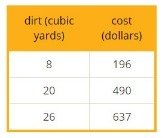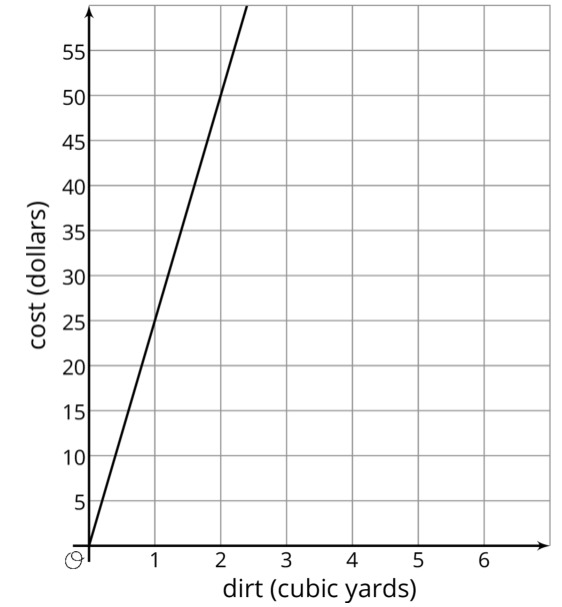How much would EZ Excavation charge to haul 40 cubic yards of dirt?

Do not include units ($) in your answer. copied for free from openupresources.org Type your answer below as a number (example: 5, 3.1, 4 1/2, or 3/2): Part B) Explain or show your reasoning. Type your answer below: Part C) How much would Happy Hauling Service charge to haul 40 cubic yards of dirt? Do not include units ($) in your answer.

Type your answer below as a number (example: 5, 3.1, 4 1/2, or 3/2):
Part D)

Part E)

Calculate the rate of change for EZ Excavation.

Do not include units ($/cubic yd) in your answer. Type your answer below as a number (example: 5, 3.1, 4 1/2, or 3/2): Part F) Calculate the rate of change for Happy Hauling Service. Do not include units ($/cubic yd) in your answer.

Type your answer below as a number (example: 5, 3.1, 4 1/2, or 3/2):
Part G)

What do the rates of change mean for each company?

Part H)

If the contractor has 40 cubic yards of dirt to haul and a budget of \$1000, which hauling company should she hire?

Select one:
Part I)

Part A)

Andre and Priya are tracking the number of steps they walk. Andre records that he can walk 6000 steps in 50 minutes. Priya writes the equation 118x, where y is the number of steps and x is the number of minutes she walks, to describe her step rate. This week, Andre and Priya each walk for a total of 5 hours. Who walks more steps?

Select one:
Part B)

How many steps more?

Type your answer below as a number (example: 5, 3.1, 4 1/2, or 3/2):
Part A)

Find the coordinates of point D in the diagram below: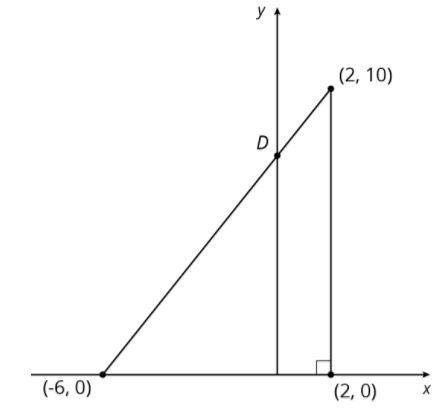(x,y)

with no spaces

If x or y is a mixed number, write it in fraction form

Ex: If the answer is x=3 and y=, then write "(3,5/2)"

Part B)

Find the coordinates of point D in the diagram below: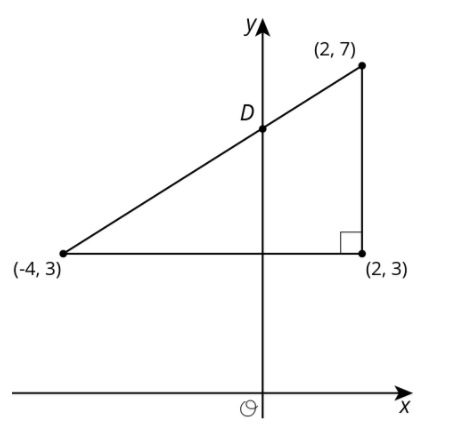(x,y)
with no spaces

If x or y is a mixed number, write it in fraction form

Ex: If the answer is x=3 and y=, then write "(3,5/2)".

Part A)

Solve each equation.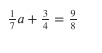a= ________

Part B)

Solve each equation.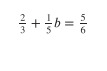b= ________

Part C)

Solve each equation.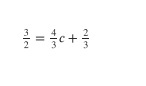c= ________

Part D)

Solve each equation.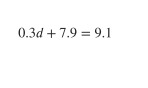d= ________

Type your answer below as a number (example: 5, 3.1, 4 1/2, or 3/2):
Part E)

Solve each equation.